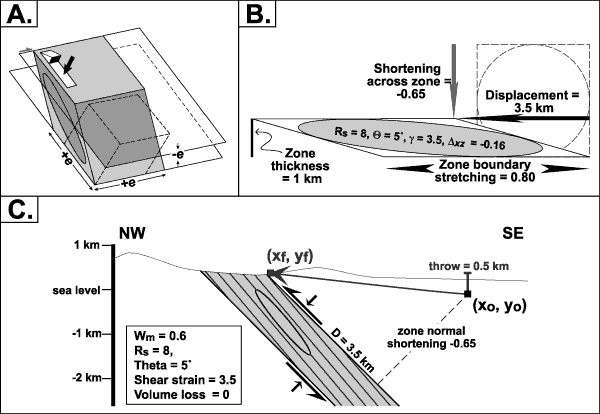Figure 10. A, Idealized finite deformation for Blue Ridge high-strain zones characterized by a weak triclinic symmetry, general shear, and flattening strain (elongation in two directions, shortening in one). Ellipse represents XZ section of the three-dimensional finite strain ellipsoid. B, Kinematic model for deformation in the Quaker Run high-strain zone. The box and circle represent basement in undeformed state that is transformed into parallelogram and ellipse during homogeneous deformation. The model assumes a monoclinic deformation symmetry, general shear (Wm= 0.6), flattening strain (K=0.6), and no volume loss (delta=0). The zone is ~1 km (0.6 mi) thick, the mean XZ strain ratio (RXZ) is 8:1 (based on strain estimates from quartz grain shapes), shear strain (gamma) is 3.5, and the orientation between the foliation and high-strain zone boundary (theta) is 5°. There is a sectional area loss (deltaXZ =-0.16) because of flattening strain with no volume change. Displacement across the zone is 3.5 km (2.2 mi) and is accompanied by 80 percent stretching parallel to the zone boundary and 65 percent shortening across the zone. C, Displacement, shortening, and tectonic throw across the Quaker Run high-strain zone viewed in cross section, based on deformation parameters illustrated in figure 10B. Rock exposed at (Xf,Yf) originated at (Xo,Yo) prior to deformation.

U.S. Department of the Interior, U.S. Geological Survey
URL: https:// pubs.usgs.gov /circ/2004/1264/html/trip2/fig10.html